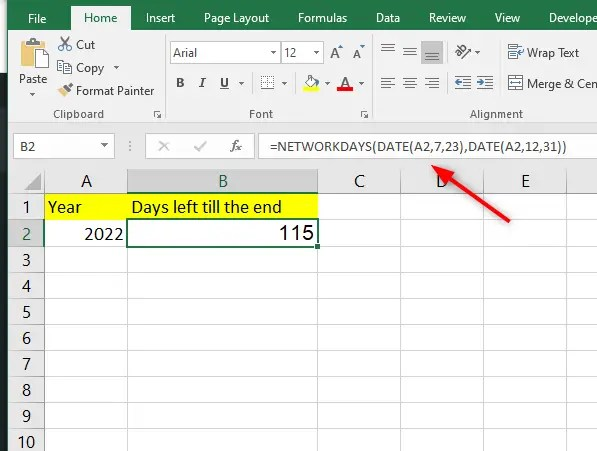# How to calculate days in Excel till the end

In certain circumstances, you may want to calculate the days in Excel till the end  of a particular month, date, or year. This mostly applies while working with projects in Excel. Calculating the days till the end enables you to keep up with commitments and beat deadlines.

This tutorial discusses the different methods you can use to calculate days till the end in Excel. Let’s get started.

## Calculate days till the end using the DAYS function in Excel

Here, we use the formula;

=DAYS (End date, TODAY ())

Where;

TODAY is the function that returns the date of the present day.

DAYS function subtracts the end date from the resent day date.

You can use this formula to count down the days to an important event or milestone or until the expiration of a subscription.

To use this formula, here is what to do.

1. In an empty cell next to your data, type in the formula above. In place of the end date, specify the cell that contains the end date.

2. Press Enter key on your keyboard.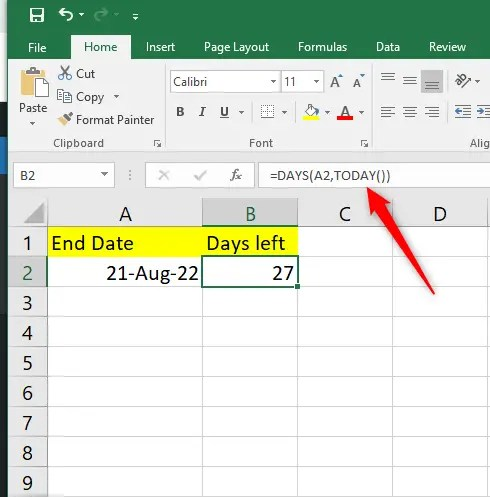Make sure the cell is formatted as general to have the correct data.

3. Click on the Home tab of the main menu ribbon.

4. In the Numbers group, click on the drop-down arrow and select General.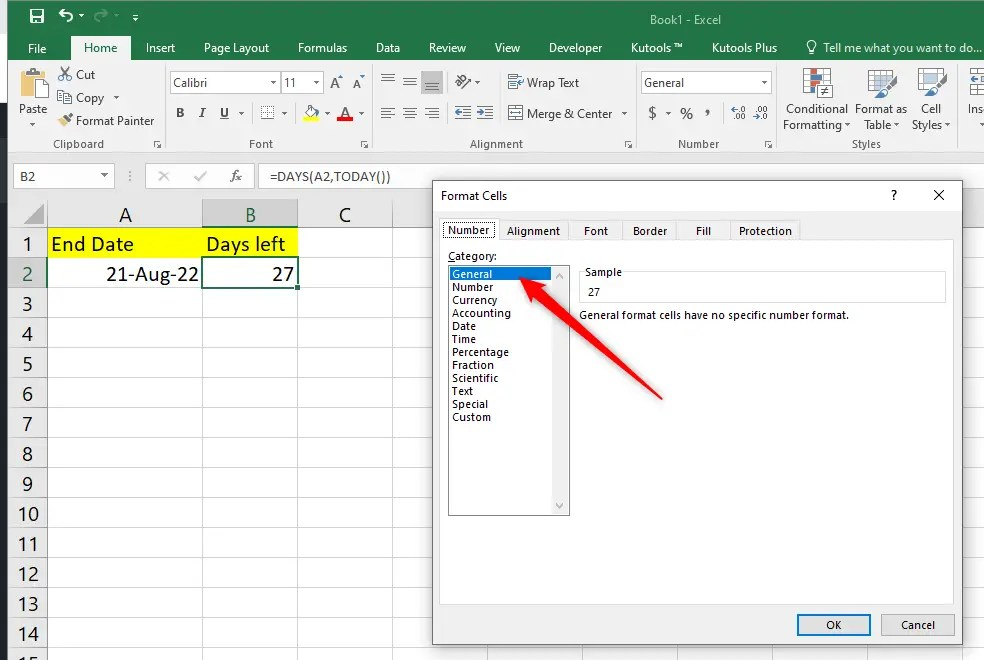5. Afterward, you will notice that the number format is now a numerical value representing the number of remaining days for your project or until the end.

6. Apply this formula to other cells by dragging the fill handle.

## Calculating days till end using subtraction and TODAY function

One of the easiest and quickest ways to calculate days till the end is subtraction. Here is how you can do that.

• Write down the formula below in an empty cell where you want your results.

=MAX (0, end date-TODAY ()

Whereby;

TODAY function represents the date of the present day. The arithmetic expression end date-TODAY () will subtract your end date from the present-day date.

MAX function will return a subtracted value if it’s positive. If the present-day date exceeds the end date, the MAX function will return zero instead of a negative value.• Press You will get your result in Date format.
• Click on the Home tab and go to the Number group to change the Number format into a General format to get the numerical value of how many days from the present are left until the deadline of the project.
• Next, get the remaining days of the project or if you are dealing with multiple projects, drag the fill handle to apply the formula.

## Calculating the days left in a month

Suppose the deadline of your project is at the end of the month, you can use the EOMONTH function to calculate the days till the end month.

Use the formula;

=EOMONTH (End date, 0)-End date

EOMONTH function returns the last date of the month from the end date.

The last day of the month is subtracted from the end date to determine how many days are left in that month.

1. Write down your formula in an empty cell next to your data.2. Press the Enter key on your keyboard.

3. Go to the Number format group, click on the drop-down arrow and select general4. Press OK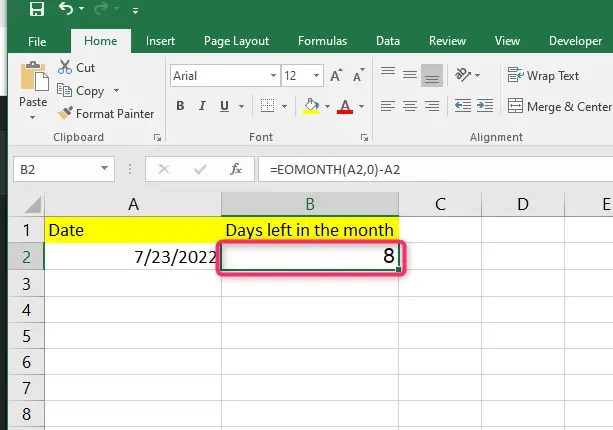Excel template to calculate the days left till the End

## How to calculate Workdays left in the month using Excel

Workdays consist of Monday to Friday. EOMONTH counts all the days, including weekends.  You can combine the EOMONTH function and NETWORKDAYS function to find workdays left in the month.

1. Write the current date on one cell. For example, in cell A2

2. Enter the following formula on an empty cell=NETWORKDAYS(A2,EOMONTH(A2,0))

3. Press okay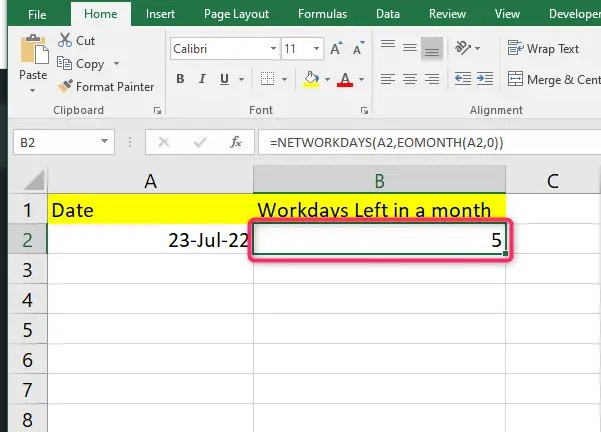## How to calculate the number of days left in a Year

You can easily count the number of days till the end of a year using a combination of the year and date function. Follow these simple steps.

1. Write a date on one cell.

2. Enter the following formula on another empty cell=DATE(YEAR(A2),12,31)-A2

3. Press Enter and the number of days left till the end of the year will populate### How to calculate the number of days till the end of the year from today

If you wish to count the number of days left till the end of the year from today, you need to combine years and today’s function. Follow these simple steps.

Write the following formula in an empty cell and press enter.

=DATE(YEAR(TODAY()),12,31)-TODAY()## How to calculate working days till the end of the year

Counting work days left in a year can be very useful, especially if you want to plan your days off well. If you count manually, you will spend hours and might make some mistakes. Luckily Excel comes with functions that can count these days easily for you. Follow these simple steps.

We are going to combine two functions, Networkdays() and date()

1. Enter the current year in a cell; let’s say A2

2. Enter the following formula in another cell and press enter